# Python 字符串详解之其他功能下

``l = ['刘备', '曹操', '孙权', '袁绍']``

``````l = ['刘备', '曹操', '孙权', '袁绍']
print('-'.join(l))``````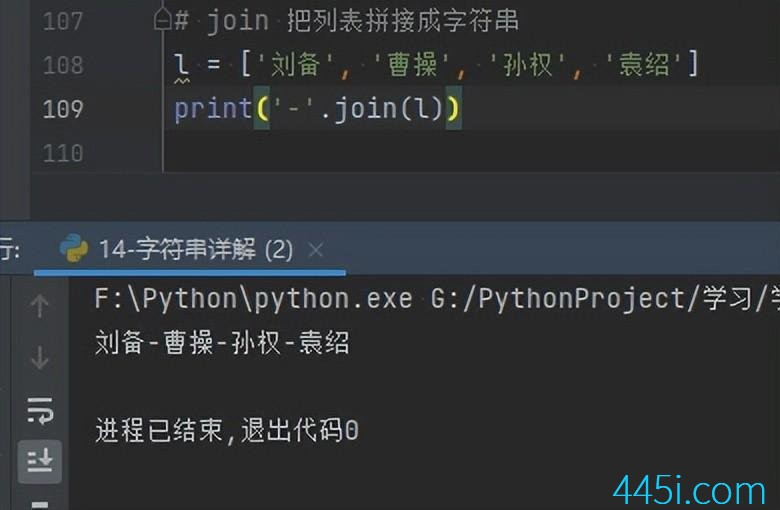``````l = ['刘备', '曹操', '孙权', '袁绍'，1]
print('-'.join(l))``````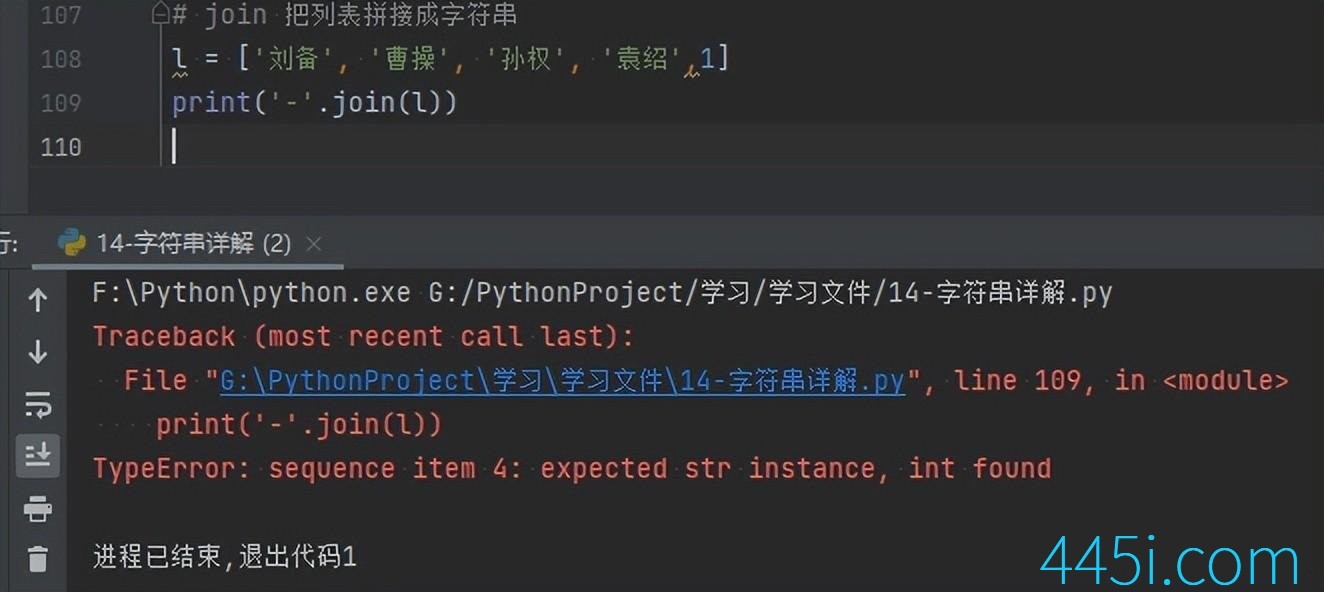``names = '李白-杜甫-白居易-陶渊明'``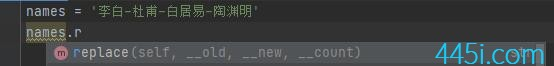``````names = '李白-杜甫-白居易-陶渊明'
print(names.replace('-', '*'))``````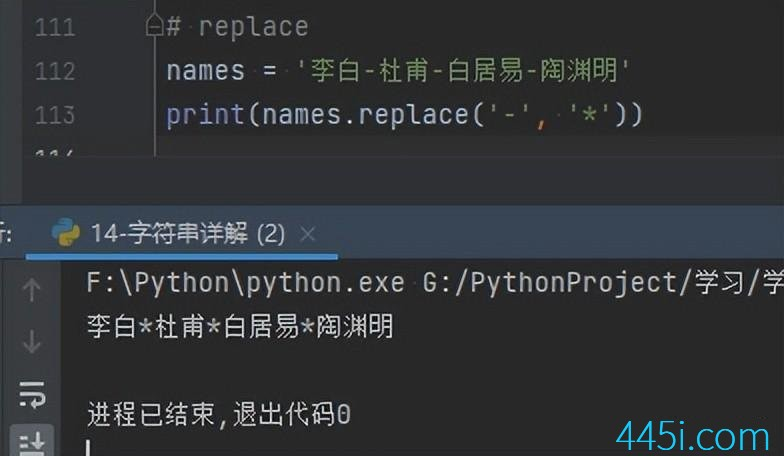``````names = '李白-杜甫-白居易-陶渊明'
print(names.replace('-', '*'))
print(names.replace('李白', '诗仙'))``````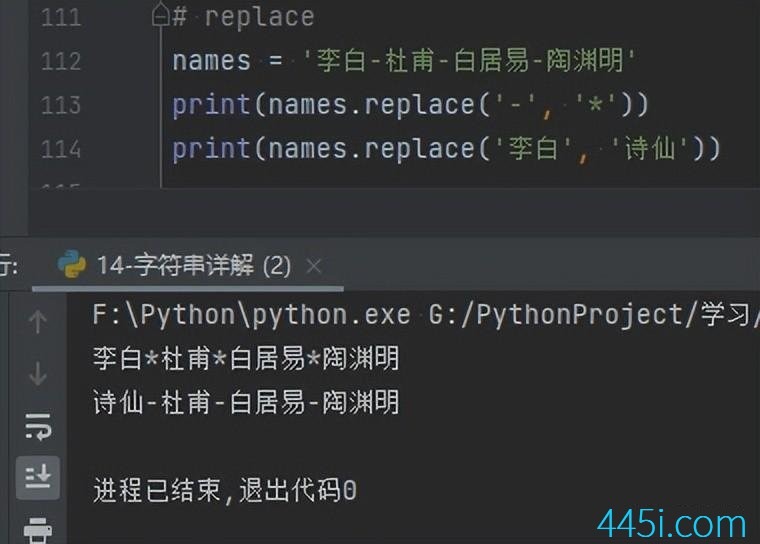``````print('84'.isdigit())
print('7.a4'.isdigit())``````### 觉得文章有用就打赏一下文章作者

#### 支付宝扫一扫打赏#### 微信扫一扫打赏